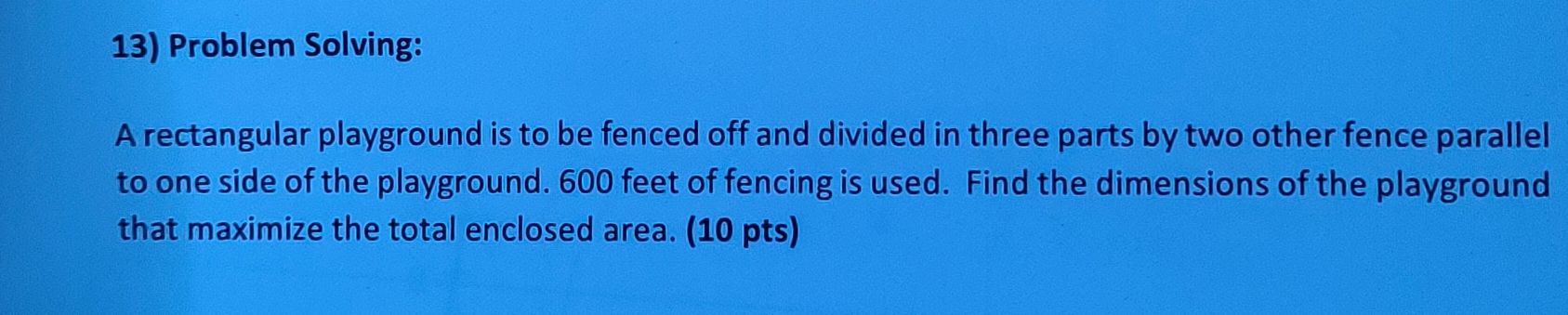### ¿Todavía tienes preguntas de matemáticas?

Pregunte a nuestros tutores expertos
Algebra
Pregunta13) Problem Solving: A rectangular playground is to be fenced off and divided in three parts by two other fence parallel to one side of the playground. $$600$$ feet of fencing is used. Find the dimensions of the playground that maximize the total enclosed area. ( $$10$$ pts)

$$A= x(300- 2x)= -2x^2+ 300x\\A_{\max}= A_{x= 75}= 11250$$